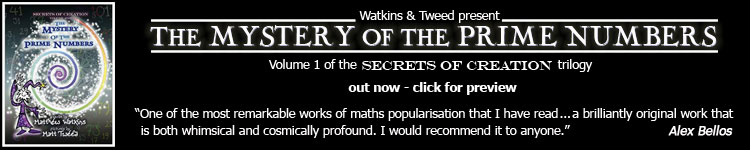## prime numbers: FAQ and resources

further resources

the distribution of prime numbers (elementary, visually-oriented presentation)

quotations about the mysterious qualities of the prime distribution

the prime number theorem (proof outline and additional notes)

the Riemann Hypothesis FAQ and resources

Riemann's zeta function resources

D. Rockmore, "Chance in the Primes" [part 1]  [part 2]  [part 3]
This excellent and thorough article is intended as a commentary to supplement the first half of a popular talk on the Riemann Hypothesis given by Peter Sarnak at a 1998 MSRI conference (a video recording of which is available here).

the functional equation of the Riemann zeta function and related issues

D. Bump's lecture notes on the distribution of the Riemann zeta function's nontrivial zeros

summary of Ilan Vardi's (excellent) "Introduction to Analytic Number Theory"

mystery      new      number theory and physics archive      search      home